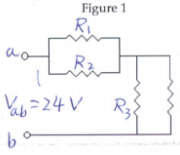# Problem: The resistors in the circuit shown in Figure 1 each have a resistance of 700 Ω.Find (a) the equivalent resistance of the circuit and (b) the current flowing through each resistor R if the input voltage is 24 volts.

###### FREE Expert Solution
93% (215 ratings)
###### Problem Details

The resistors in the circuit shown in Figure 1 each have a resistance of 700 Ω.Find (a) the equivalent resistance of the circuit and (b) the current flowing through each resistor R if the input voltage is 24 volts.